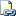# IS NULL (Transact-SQL)

Determines whether a specified expression is NULL.Transact-SQL Syntax Conventions

## Syntax

``````expression IS [ NOT ] NULL
``````

## Arguments

• expression
Is any valid expression.

• NOT
Specifies that the Boolean result be negated. The predicate reverses its return values, returning TRUE if the value is not NULL, and FALSE if the value is NULL.

Boolean

## Return Code Values

If the value of expression is NULL, IS NULL returns TRUE; otherwise, it returns FALSE.

If the value of expression is NULL, IS NOT NULL returns FALSE; otherwise, it returns TRUE.

## Remarks

To determine whether an expression is NULL, use IS NULL or IS NOT NULL instead of comparison operators (such as = or !=). Comparison operators return UNKNOWN when either or both arguments are NULL.

## Examples

The following example returns the name and the weight for all products for which either the weight is less than 10 pounds or the color is unknown, or NULL.

``````USE AdventureWorks2012;
GO
SELECT Name, Weight, Color
FROM Production.Product
WHERE Weight < 10.00 OR Color IS NULL
ORDER BY Name;
GO
``````

#### Reference

CASE (Transact-SQL)

CREATE PROCEDURE (Transact-SQL)

CREATE TABLE (Transact-SQL)

Data Types (Transact-SQL)

Expressions (Transact-SQL)

INSERT (Transact-SQL)

LIKE (Transact-SQL)

Operators (Transact-SQL)

Logical Operators (Transact-SQL)

SELECT (Transact-SQL)

sp_help (Transact-SQL)

UPDATE (Transact-SQL)

WHERE (Transact-SQL)